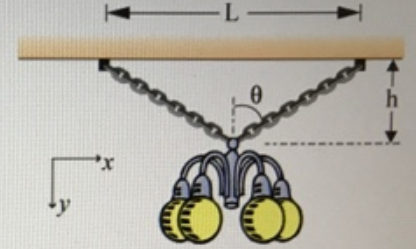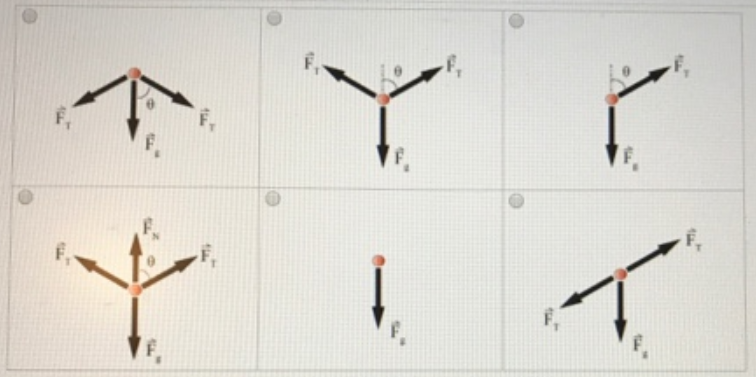# Problem: A chandelier hangs h 0.98 m down from two chains of equal length. The chains are separated from one another by a length L = 0.85 m at the ceiling. The chandelier has a mass of m = 21 kg. Randomized Variables h= 0.98 m L-0.85 m m-21 kg (a) Choose the correct Free Body Diagram given the gravitational force, F force. Fg the force exerted by the chains, FT and the normal force, FN.Part (b) What is the angle, θ in degrees, between one of the chains and the vertical where it contacts the chandelier?Part (c) Write an expression for FTy, the magnitude of the y-component of the tension in one chain, in terms of the given information and variables available in the palette.Part (d) Using your previous results, find the tension, FT in Newtons, in one chain.

###### FREE Expert Solution
99% (290 ratings)###### Problem Details

A chandelier hangs h 0.98 m down from two chains of equal length. The chains are separated from one another by a length L = 0.85 m at the ceiling. The chandelier has a mass of m = 21 kg.

Randomized Variables

h= 0.98 m

L-0.85 m

m-21 kg(a) Choose the correct Free Body Diagram given the gravitational force, F force. Fg the force exerted by the chains, FT and the normal force, FN.Part (b) What is the angle, θ in degrees, between one of the chains and the vertical where it contacts the chandelier?

Part (c) Write an expression for FTy, the magnitude of the y-component of the tension in one chain, in terms of the given information and variables available in the palette.

Part (d) Using your previous results, find the tension, FT in Newtons, in one chain.